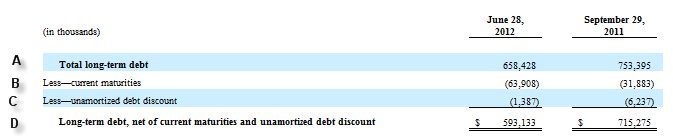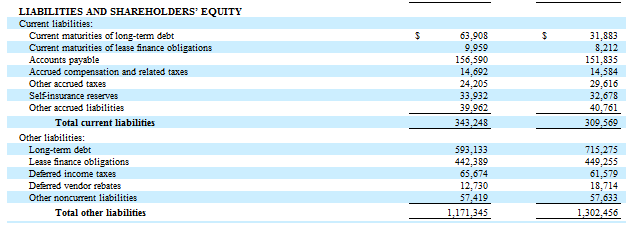#### Issue

In some disclosures, the nature of a defined calculation cannot be defined in a single calculation relationship. Below is an excerpt of the table which shows the problem.

In this case, the company does not disclose the concept – Total Long-Term Debt NET of unamortized discount, gross.The balance attributes of each of these items is as follows:

A – 658,428 (Credit)

B – 63,908 (Credit)

C – 1,387 (Debit)

D – 593,133 (Credit)

Below is the balance sheet screenshot (for additional info) so you can see how the carrying values for Long-Term Debt noncurrent and current portion of LTD tie back to the B/S:The financial statements show an effective calculation; however, there is no way to express it in XBRL even if the calculation is reversed.  This calculation, however, could be expressed if there was an interim aggregation point, but the company does not report this.

#### Recommendation

The calculation can be expressed if calculations are defined for a common parent element that is not defined in the instance document.

Long term debt (\$657,041 CR) = (D) Long term debt excluding Current Maturities of \$593,133 (CR) + (B) Current Maturities of \$63,908 (CR)Long Term Debt (657,041 CR) = (A) Long term debt, Gross of \$658,428 (CR) – (C) Unamortized Debt Discount of \$1,387 (DR)Due to EFM 6.15.3, one of the calculation relationships would need to be included in a distinct calculation linkbase.

The value for the parent element in each calculation is not reported.  So the element would be in the calculation and presentation but would not be tagged with a value. Therefore, no XBRL 2.1 calculation inconsistency would result.

Depending on interpretation and EDGAR system logic of EFM 6.14.5, it may require including the unreported parent element in the presentation link.  Since there is no value for this element, it will not impact rendering.

The Best Practice Committee does not consider this case to fall within the definition of a required calculation.  The method to express the calculation is included as guidance if the filer wants to capture the calculation relationship represented in the filing.